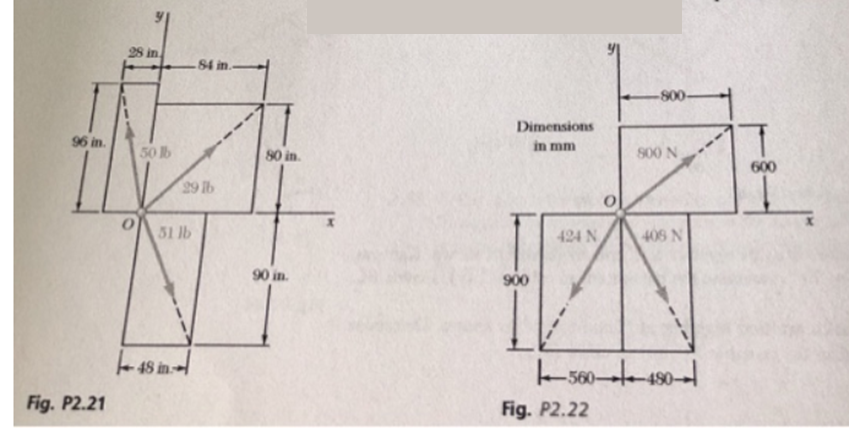# Problem: Determine the x and y components of each of the forces shown.

###### FREE Expert Solution

2D vector Direction/Components:

$\overline{){\mathbf{tan}}{\mathbf{\theta }}{\mathbf{=}}\frac{\mathbf{y}}{\mathbf{x}}}$

29 lb force

θ = tan-1 (y/x) = tan-1 (80/84) = 43.6°

Fx = Fcosθ = 29cos43.6 = 21.0 lb

Fy = Fsinθ = 29sin43.6 = 20.0 lb

100% (409 ratings)###### Problem Details

Determine the x and y components of each of the forces shown.